# MP Board Class 6th Maths Solutions Chapter 9 Data Handling Ex 9.4

## MP Board Class 6th Maths Solutions Chapter 9 Data Handling Ex 9.4

Question 1.
A survey of 120 school students was done to find which activity they prefer to do in their free time.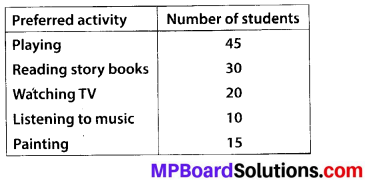Draw a bar graph to illustrate the above data taking scale of 1 unit length = 5 students. Which activity is preferred by most of the students other than playing?
Solution: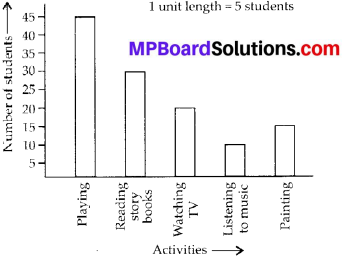Reading story books is preferred by most of the students other than playing.

Question 2.
The number of Mathematics books sold by a shopkeeper on six consecutive days is shown below: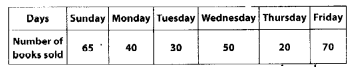Draw a bar graph to represent the above information choosing the scale of your choice.
Solution: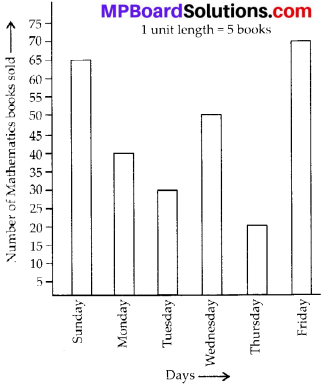Question 3.
Following table shows the number of bicycles manufactured in a factory during the years 1998 to 2002. Illustrate this data using a bar graph. Choose a scale of your choice.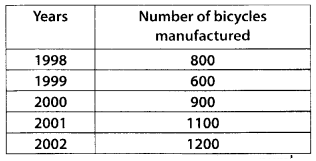(a) In which year were the maximum number of bicycles manufactured?
(b) In which year were the minimum number of bicycles manufactured?
Solution: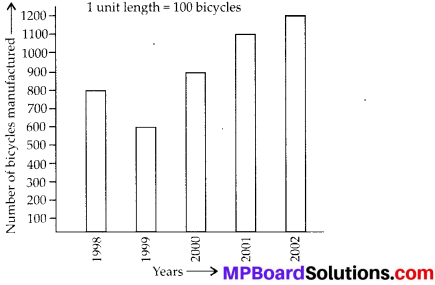(a) In 2002, the maximum number of bicycles were manufactured.
(b) In 1999, the minimum number of bicycles were manufactured.Question 4.
Number of persons in various age groups in a town is given in the following table.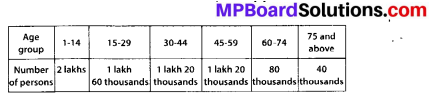Draw a bar graph to represent the above information and answer the following questions, (take 1 unit length = 20 thousands)
(a) Which two age groups have same Population?
(b) All persons in the age group of 60 and above are called senior citizens. How many senior citizens are there in the town?
Solution: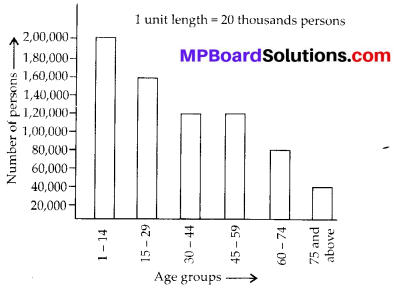(a) Age groups 30 – 44 and 45 – 59 have same population.
(b) 80,000 + 40,000 = 1,20,000 senior citizens are there in the town.